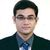# QlikView App Dev

Discussion Board for collaboration related to QlikView App Development.

Announcements
Join this live chat April 6, 10AM EST - QlikView to Qlik Sense REGISTER
cancel
Showing results for
Did you mean:Not applicable

## Check the number of rows from QVD for selected date range

Hi,

My QVD holds 2 years of data. And total row count 9000001. I am looking for number of rows from a QVD starting with two date range i.e. Minimum date and maximum date. But I am getting data for one date. Please advise.

Let vMaxdate= date#('31/05/2014','DD/MM/YYYY');

Let vMindate=date#('31/05/2016','DD/MM/YYYY');

Test Table:

FROM

(qvd)

WHERE  TRANSACTION_DATE >= '\$(vMaxdate)' and TRANSACTION_DATE <= '\$(vMindate)';

LET a = NoOfRows('Test Table');

Rohit

1 Solution

Accepted SolutionsCreator III

Hi Rohit,

Make sure the TRANSACTION_DATE field is properly formatted to date type, remove the quotes around the variables in the where clause and use \$(#vMindate) for the values to be interpreted as numeric.

Also, the WHERE clause logic is wrong as no date can be in the same time grater than the max date and less than min date. It should be:

WHERE TRANSACTION_DATE >= \$(#vMindate) AND TRANSACTION_DATE<= \$(#vMaxdate)

Let vMaxdate= date#('31/05/2014','DD/MM/YYYY');

Let vMindate=date#('31/05/2016','DD/MM/YYYY');

Test Table:

FROM

(qvd)

WHERE  TRANSACTION_DATE >= \$(#vMindate) and TRANSACTION_DATE <= \$(#vMaxdate);

LET a = NoOfRows('Test Table');

Regards,

David

5 RepliesMVP

Hi,

Try this.

Let vMaxdate= num(Date(date#('31/05/2014','DD/MM/YYYY')));

Let vMindate=num(Date(date#('31/05/2016','DD/MM/YYYY')));

Test_Table:

FROM

(qvd)

WHERE  TRANSACTION_DATE >= '\$(vMindate)' and TRANSACTION_DATE <= '\$(vMaxdate)';

LET a = NoOfRows('Test_Table');

Regards,

Kaushik Solanki

Regards,
KaushikNot applicable
Author

Hi Kaushik,

I am getting below result:

vMaxdate = 41790

vMindate = 42521

And the Test_Table having 0 rows fetched after query execution.

Test_Table << MasterDatacsv 0 Lines fetched

a = 0  <-- it hold value as 0 record

Thanks,

RohitCreator III

Hi Rohit,

Make sure the TRANSACTION_DATE field is properly formatted to date type, remove the quotes around the variables in the where clause and use \$(#vMindate) for the values to be interpreted as numeric.

Also, the WHERE clause logic is wrong as no date can be in the same time grater than the max date and less than min date. It should be:

WHERE TRANSACTION_DATE >= \$(#vMindate) AND TRANSACTION_DATE<= \$(#vMaxdate)

Let vMaxdate= date#('31/05/2014','DD/MM/YYYY');

Let vMindate=date#('31/05/2016','DD/MM/YYYY');

Test Table:

FROM

(qvd)

WHERE  TRANSACTION_DATE >= \$(#vMindate) and TRANSACTION_DATE <= \$(#vMaxdate);

LET a = NoOfRows('Test Table');

Regards,

DavidMVP

Hi,

I got it.

I changes the sign of the where condition, but forgot to swipe the variables.

Change the vMaxdate to vMindate and vice versa.

so your Min Date should be 41790 and Max Date should be 42521

Regards,

Kaushik Solanki

Regards,
Kaushik